How Cheenta works to ensure student success?
Explore the Back-Story

# NMTC 2023 Stage II - Kaprekar (Grade 7 & 8) - Problems and Solutions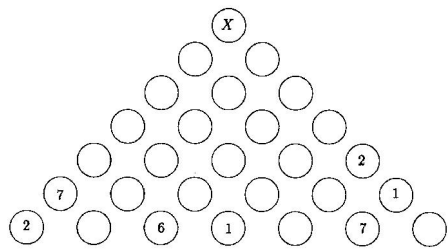###### Problem 1

If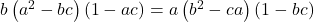where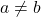and, prove that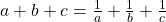###### Problem 2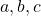are three distinct positive integers. Show that among the numbers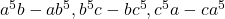there must be one which is divisible by 8 .

###### Problem 3

There are four points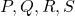on a plane such that no three of them are collinear. Can the trianglesand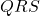be such that at least one has an interior angle less than or equal to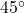? If so, how? If not, why?

###### Problem 4

A straight lineis drawn through the vertex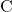of an equilateral triangle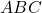, wholly lying outside the triangle.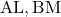are drawn perpendiculars to the straight line. Ifis the midpoint of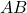, prove that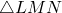is an equilateral triangle.

###### Problem 5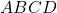is a parallelogram. Through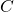, a straight line is drawn outside the parallelogram.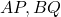and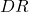are drawn perpendicular to this line Show that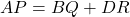. If the line throughcuts one side internally, then will the same result hold? If so prove it. If not, what is the corresponding result? Justify your answer.

###### Problem 6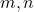are non-negative real numbers whose sum is 1 . Prove that the maximum and minimum values of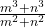are respectively 1 and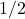.

###### Problem 7

(a) Solve for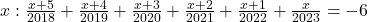(b) If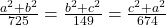and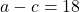, find the value of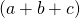.

###### Problem 8

If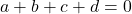, prove that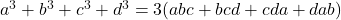###### Problem 1

Ifwhereand, prove that###### Problem 2are three distinct positive integers. Show that among the numbersthere must be one which is divisible by 8 .

###### Problem 3

There are four pointson a plane such that no three of them are collinear. Can the trianglesandbe such that at least one has an interior angle less than or equal to? If so, how? If not, why?

###### Problem 4

A straight lineis drawn through the vertexof an equilateral triangle, wholly lying outside the triangle.are drawn perpendiculars to the straight line. Ifis the midpoint of, prove thatis an equilateral triangle.

###### Problem 5is a parallelogram. Through, a straight line is drawn outside the parallelogram.andare drawn perpendicular to this line Show that. If the line throughcuts one side internally, then will the same result hold? If so prove it. If not, what is the corresponding result? Justify your answer.

###### Problem 6are non-negative real numbers whose sum is 1 . Prove that the maximum and minimum values ofare respectively 1 and.

###### Problem 7

(a) Solve for(b) Ifand, find the value of.

###### Problem 8

If, prove thatThis site uses Akismet to reduce spam. Learn how your comment data is processed.

### Knowledge Partner